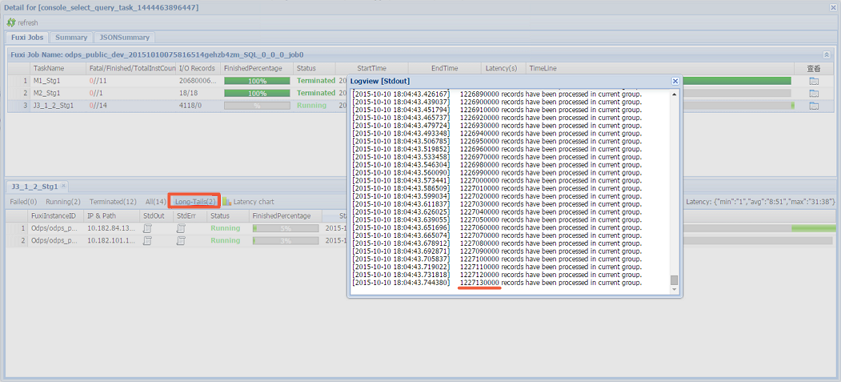## 数据倾斜优化

• Join操作导致的数据倾斜
Join操作导致数据倾斜的原因是Join on的Key分布不均匀。假设大表A和小表B执行Join操作，运行如下语句。
``SELECT * FROM A JOIN B ON A.value = B.value;``
复制该语句的Logview链接并打开，双击执行Join操作的作业，可以看到此时在[Long-tails]区域存在长尾现象，表示数据已经倾斜了。您可通过如下方法进行优化：
• 由于表B是个小表并且没有超过512 MB，您可将上述语句优化为MapJoin语句后执行，语句如下。
``SELECT /* + MAPJOIN(B) */ * FROM A JOIN B ON A.value = B.value;``
• 将倾斜的Key用单独的逻辑来处理。假设两边的Key中有大量NULL数据导致了倾斜，则需要在Join前先过滤掉NULL数据或者补上随机数，然后再进行Join，示例如下。
``SELECT * FROM A JOIN B ON CASE WHEN A.value IS NULL THEN CONCAT('value',RAND() ) ELSE A.value END = B.value;``
在实际场景中，如果您发现已经数据倾斜，但无法获取导致数据倾斜的Key信息，可以使用如下方法查看数据倾斜。
``````--执行如下语句产生数据倾斜。
SELECT * FROM a JOIN b ON a.key=b.key;
--您可以执行如下SQL，查看Key的分布，判断执行Join操作时是否会有数据倾斜。
SELECT left.key, left.cnt * right.cnt FROM
(select key, count(*) AS cnt FROM a GROUP BY key) LEFT
JOIN
(SELECT key, COUNT(*) AS cnt FROM b GROUP BY key) RIGHT
ON left.key=right.key;``````
• Group By倾斜

造成Group By倾斜的原因是Group By的Key分布不均匀。

假设表A内有两个字段（Key, Value），表内的数据量较大且Key值分布不均匀，运行语句如下所示。
``SELECT key, COUNT(value) FROM A GROUP BY key;``

当表中的数据足够大时，您会在Logview中发现长尾现象。解决此问题，您需要在执行SQL前设置防倾斜的参数，设置语句为`set odps.sql.groupby.skewindata=true`

• 错误使用动态分区造成的数据倾斜
动态分区SQL时，在MaxCompute中会默认增加一个Reduce，用来将相同分区的数据合并在一起。此操作可以：
• 减少MaxCompute系统产生的小文件，使后续处理更快速。
• 避免一个Worker输出文件很多时占用内存过大。
如果引入的Reduce导致分区数据倾斜，则会发生长尾。因为相同的数据最多只会有10个Worker处理，所以数据量大，则会发生长尾，示例如下。
``INSERT OVERWRITE TABLE A2 PARTITION(dt) SELECT SPLIT_PART(value,'\t',1) AS field1, SPLIT_PART(value,'\t',2) AS field2, dt FROM A WHERE dt='20151010';``
这种情况下，不建议使用动态分区，优化语句如下。
``````INSERT OVERWRITE TABLE A2 PARTITION (dt='20151010')
SELECT
SPLIT_PART(value,'\t',1) as field1,
SPLIT_PART(value,'\t',2) as field2
FROM A
WHERE dt='20151010';``````

## 窗口函数优化

• 窗口函数在OVER关键字后面要完全相同，要有相同的分组和排序条件。
• 多个窗口函数在同一层SQL中执行。

``````SELECT
RANK()OVER(PARTITION BY A ORDER BY B desc) AS RANK,
ROW_NUMBER()OVER(PARTITION BY A ORDER BY B desc) AS row_num
FROM MyTable;``````

## 子查询优化

``SELECT * FROM table_a a WHERE a.col1 IN (SELECT col1 FROM table_b b WHERE xxx);``

``SELECT a.* FROM table_a a JOIN (SELECT DISTINCT col1 FROM table_b b WHERE xxx) c ON (a.col1 = c.col1)``

• 如果没有使用DISTINCT关键字，而子查询表c返回的结果中有相同的col1的值，可能会导致a表的结果数变多。
• DISTINCT关键字会导致查询在同一个Worker中执行。如果子查询数据量较大，会导致查询比较慢。
• 如果业务上已经确保子查询中col1列值无重复，您可以删除DISTINCT关键字，以提高性能。

## Join语句优化

• 主表的分区限制条件可以写在WHERE子句中（最好先用子查询过滤）。
• 主表的WHERE子句建议写在SQL语句最后。
• 从表分区限制条件不要写在WHERE子句中，建议写在ON条件或者子查询中。

``````SELECT * FROM A JOIN (SELECT * FROM B WHERE dt=20150301)B ON B.id=A.id WHERE A.dt=20150301；
SELECT * FROM A JOIN B ON B.id=A.id WHERE B.dt=20150301；--不建议使用。此语句会先执行Join操作后进行分区裁剪，导致数据量变大，性能下降。
SELECT * FROM (SELECT * FROM A WHERE dt=20150301)A JOIN (SELECT * FROM B WHERE dt=20150301)B ON B.id=A.id；``````